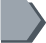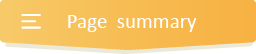Your browser does not support JavaScript!

#Mixed Operations Word Problems### Basic math operations and their key words.

Apart from carefully reading a given word problem to understand, you equally need to sort out key words or phrases that will indicate a particular operation. Thus, below a table of some basic math operations and their key words.

 Addition Subtraction Multiplication Division sum less than times split plus more than total each and decrease of cut total reduce per equal pieces increase difference as much average more remain twice every raise minus by out of both dropped area ratio combined near volume shared altogether balance product quotient

divisibility rules, division facts up to 12, dividing multi digit numbers by 1 digit, dividing whole numbers ending in zeroes, dividing 2 digit numbers and 3 digit numbers by 2 digit number, dividing 4 digit numbers by 2 digit numbers word problems grade 5, dividing decimals; fractions, etc.

•